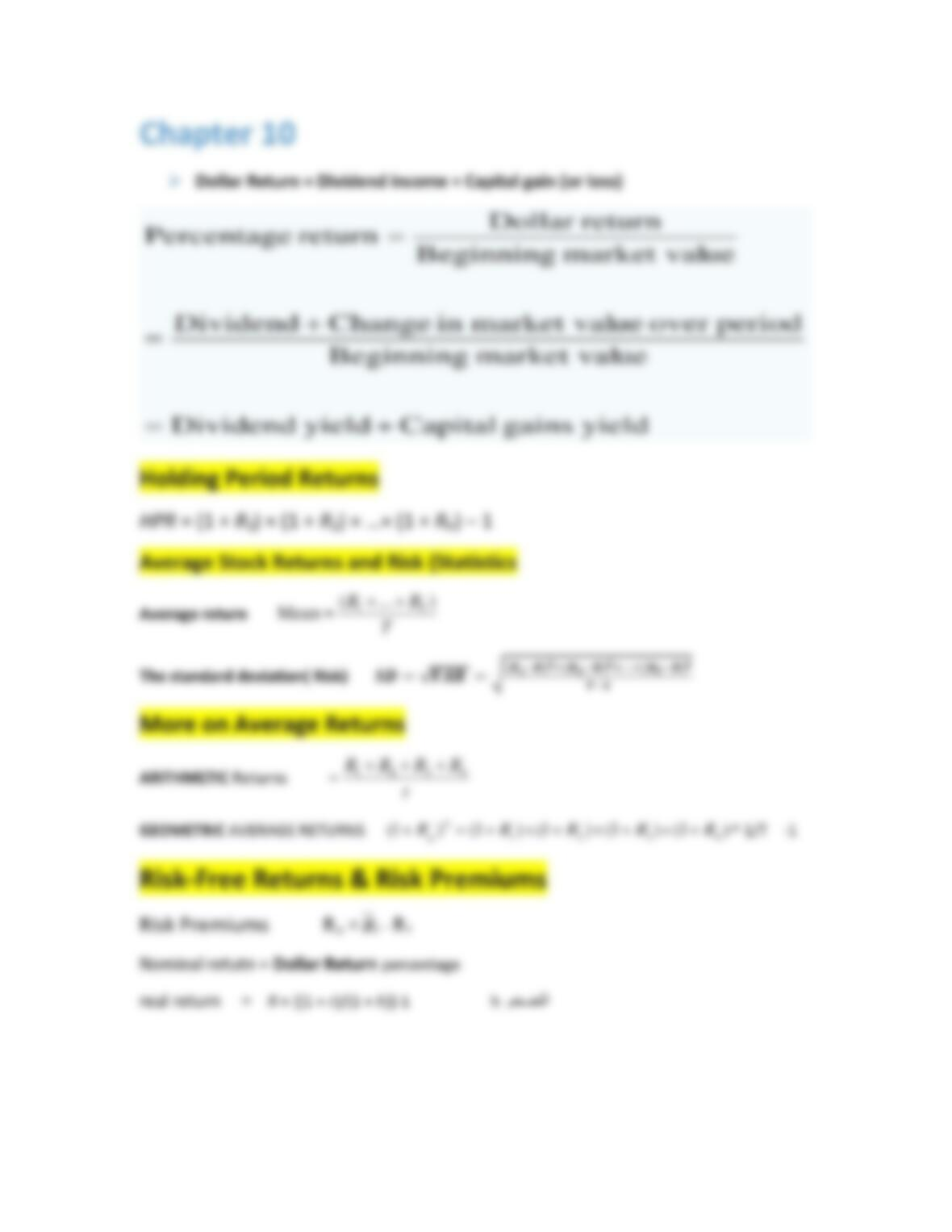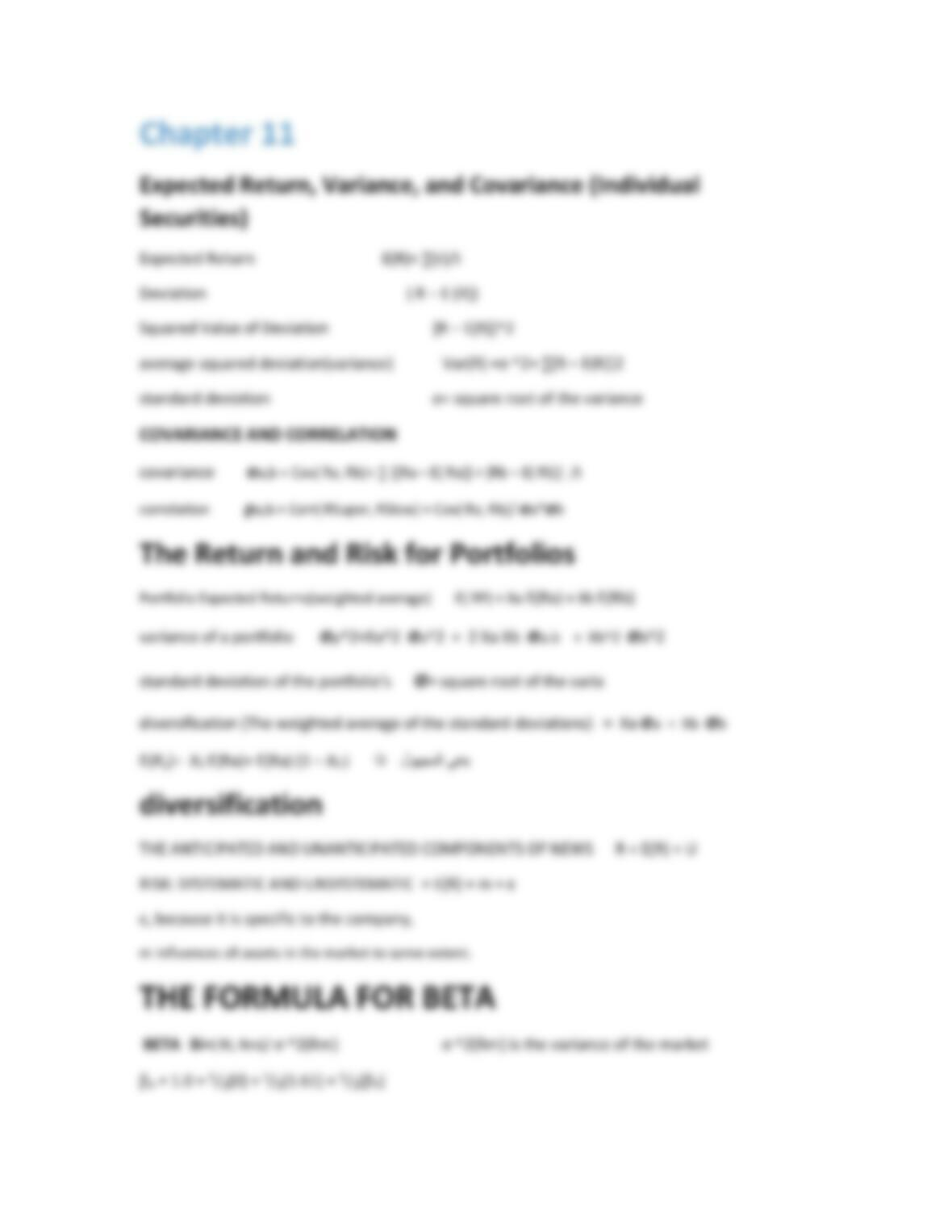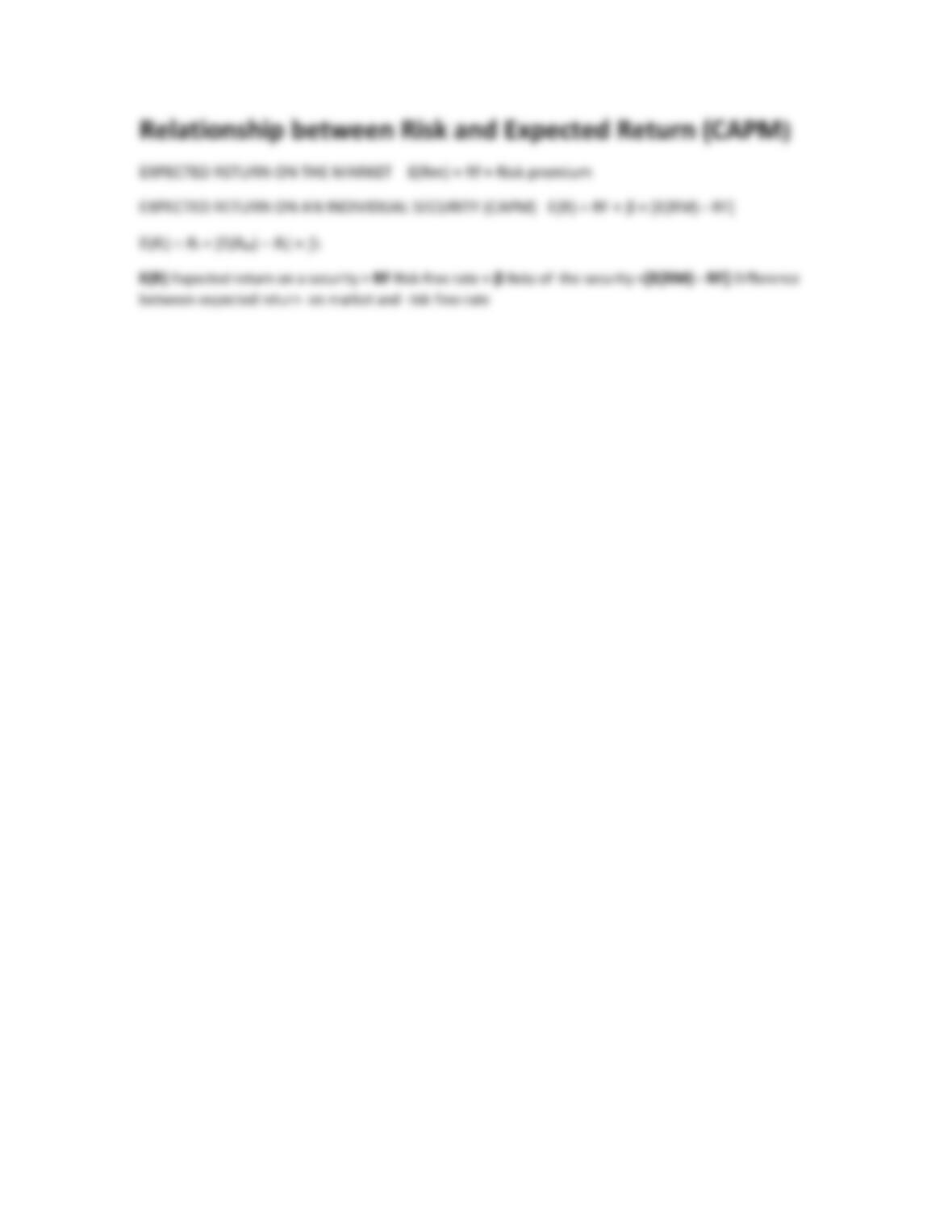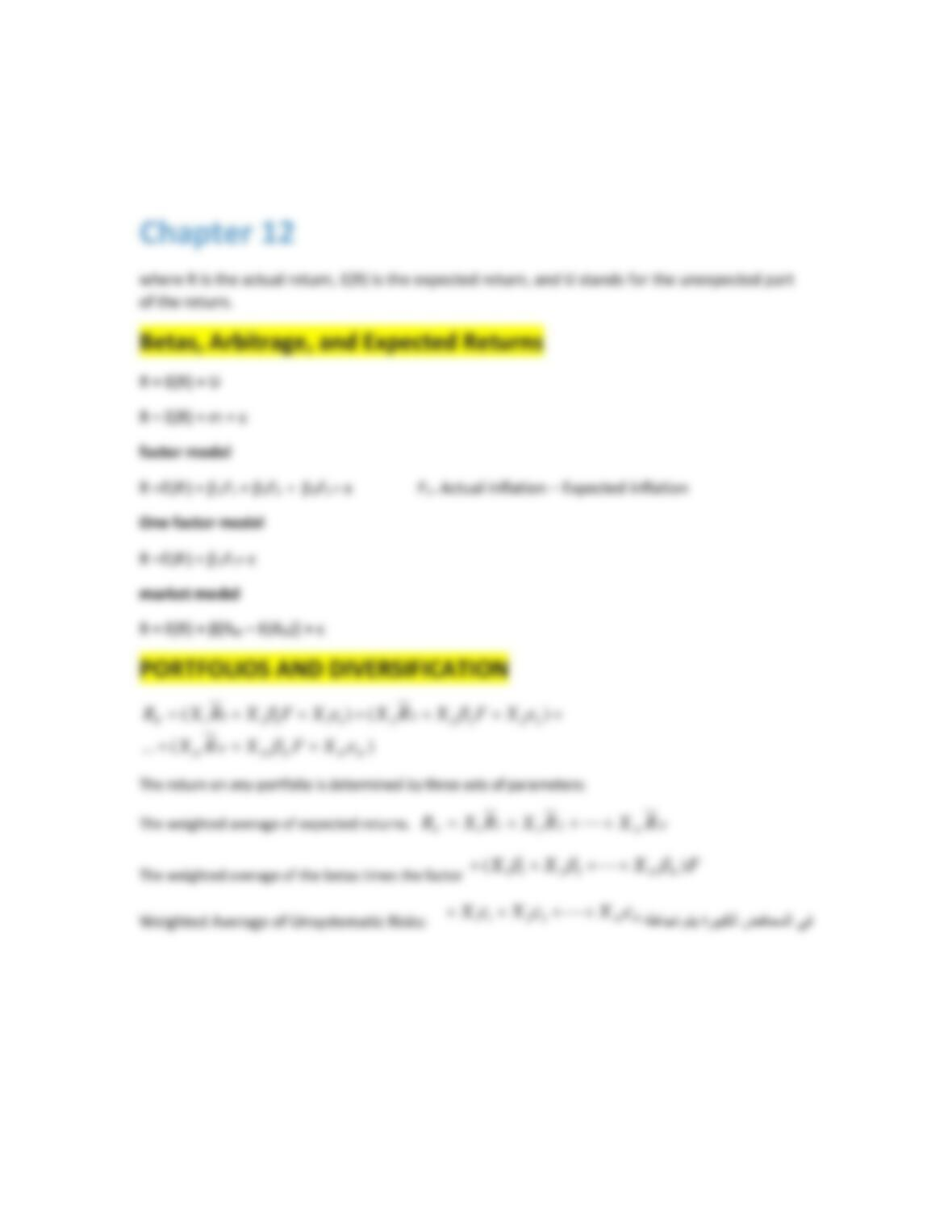Type
Essay
Pages
6 pages
Word Count
690 words
School
Course Code
MHMOO

# The Hashemite University

January 10, 2021Chapter 4
Valuation: The One‐Period Case (one year from now)
future value FV = PV *(1+R)
Present Value PV = FV /(1+R)
Net Present Value NPV = Cost + PV
The Multiperiod Case (two years from now and more)
Future Value FV = PV × (1 + r)t
Present Value PV = FV/(1 + r)t
Net Present Value NPV = −Co +( C1/ 1 + r) +( C2/( 1 + r)^2)
Find the variables
r = (FV/PV)1/t 1
t = ln(FV/PV)/ln(1 + r)
Compounding Periods occur more frequently than once a year (semiannually,
quarterly, monthly, daily)
future value (annual percentage rate ) Co*(1+R/m)^m
Effective Annual Rate EAR = [1 + (APR/m)]m 1
APR = m[(1 + EAR)1/m 1]
EAR with continuous compounding EAR = er 1
COMPOUNDING OVER MANY YEARS
future value FV = PV(1+r/m)^mt
CONTINUOUS COMPOUNDING FV = PVertSimplifications
Perpetuity PV =C/R
Growing perpetuity PV = C /r g 1.The numerator 2.The discount rate and the growth rate3. The timing assumption
Annuity PVA = C({1 [1/(1 + r)]t}/r) 4Trick باتكلا رخا لوادجلاب دوجوم a.2
FV= C({ [(1 + r)]t -1}/r)
Growing annuity PV = C{[1/(r g)] [1/(r g)] × [(1 + g)/(1 + r)]t}
Loan Amortization
Chapter 5
Payback period = 1 + (C0 C1 )/ C3
Discount Payback period= 1 + (\$15,000 10,400)/\$5,900
IRR 0 = C0 + C1/(1 + IRR) + C2/(1 + IRR)2 + C3/(1 + IRR)3
Profitability index (PI) = PV of cash flows subsequent to initial investment/Initial investmentChapter 10
Dollar Return = Dividend income + Capital gain (or loss)
Holding Period Returns
HPR = (1 + R1) × (1 + R2) × …× (1 + Rn) 1
Average Stock Returns and Risk )Statistics
Average return
T
RR T)...(
=Mean 1
yield gains Capitalyield Dividend
uemarket val Beginning
periodover uemarket valin ChangeDividend
uemarket val Beginning
returnDollar
return Percentage